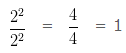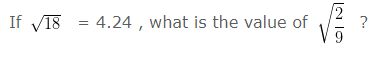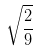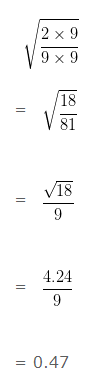# Square and Square Roots Class 8 Questions

Square and Square Roots Class 8 Questions, deals with various types of questions which are as under:-

• Finding the perfect square
• Finding the number to be multiplied to make perfect square
• Finding the square of two-digit number
• Finding the value of expression using identity
• Finding the value of square root term
• Finding the value of unknown term using identity
• Word problems on Square root

#### Square and Square Roots Class 8 Questions – Finding the perfect square

Question 1:

Which one of the following is perfect square ?

488 , 732 , 121,  3482

Solution:

The numbers which end with 2 , 3, 7 or 8 cannot be the complete squares.

Since, 488 , 732 and 3482 end with 8 , 2, 2 , therefore, they cannot be perfect square.

But, 121 can be written as

121 = 11 x 11

Hence, 121 is a perfect square.

#### Square and Square Roots Class 8 Questions – Finding the number to be multiplied to make perfect square

Question 2:

What is the least number by which 450 should be multiplied, so that the product of the two numbers would be a perfect square?

Solution:

If we find the factors of 450 , we would get 450 = 3 x 3 x 5 x 5 x 2

If we draw out the factors in pairs, we would notice that we have pairs of 3 and 5 , but 2 is the only single number, which is not in pair.

Therefore if 450 is multiplied by 2 then the product would be a complete square.

#### Square and Square Roots Class 8 Questions – Finding the square of two digit number

Question 3:

What is the value of (91)²

Solution:

We know that (a-b)² = a² + b² + 2ab

When finding the square of a larger number, we can use this equation to break the larger number into parts, whose squares can be easily calculated

We can write 91 as ( 100 – 9 )

So

91² = (100-9)²

Applying (a-b)² = a² + b² + 2ab and substituting the value of a and b, we get

= 100² + 9² – 2 x 100 x 9

= 10000 + 81 – 1800

= 10081 – 1800

= 8281

#### Square and Square Roots Class 8 Questions – Finding the value of expression using identity

Question 4:Solution:

We know that (a-b)² = a² + b² + 2ab

If we compare the above equation with the Numerator, we would find that the equation can be re-written as

= 22² – 2 x 20 x 22 + 20²

Or we can say that the given equation has value of

a = 22 and b = 20

So we can replace the numeration

22 x 22 – 2 x 20 x 22 + 20 x 20 with (22-20)² = 2²

Now putting these values in Numerator, we would getQuestion 5:

Find the value of  44² – 43² + 2²

Solution:

44² – 43² + 2²

We know that (a² – b²) = (a-b)(a+b)

a = 44 and b = 43

= ( 44 + 43 ) ( 44 – 43 ) + 4

= 87 x 1 + 4

= 87 + 4

= 91

Question 6:

The value of 555² – 554² ?

Solution:

We know that a² – b² = (a-b)(a+b)

If we compare the above equation to the problem , we find that

a = 555 and b = 554

So we can rewrite the above equation as

555² – 554² = ( 555 + 554 ) ( 555 – 554 )

= 1109 x 1

= 1109

#### Square and Square Roots Class 8 Questions – Finding the value of square root term

Question 7:Solution:

In order to solve these type of problems, we first need to equate the value which has to be solvedof the given equation in the given equation

We can write √18 = 4.24

Replacing 18 by ( 2 x 9 ) in the given equation, and dividing both the sides by 9 , we would get#### Square and Square Roots Class 8 Questions – Finding the value of unknown term using identity

Question 8:

If 42² – 40² = (12² – 10²) x Y, then the value of Y is ?

Solution:

Since,

a² – b² = (a-b) (a+b)

We can rewrite both the equation as

42² – 40² = (12² – 10²) x Y

( 42 + 40 ) x ( 42 – 40 ) = ( 12 + 10 ) x ( 12 – 10 ) x Y

82 x 2 = 22 x 2 x Y

164 = 44 x Y

Or,

Y = 164/44

Y = 41/11

#### Square and Square Roots Class 8 Questions – Word problems on Square root

Question 9:

In a conference hall of a school, 900 students were sitting in such a way that there were as many rows as there were students in a row. How many rows were there?

Solution:

Let the number of rows = Number of students in a row = x

Total number of students = Number of rows x Number of students in a row

Then

x × x = 900,

x² = 900

x=√900

= √ 3 x 3 x 5 x 5 x 2 x 2

= 3 x 5 x 2

= 30

Hence, the number of rows = Number of students in a row = 30

Question 10:

A group of People decided to collect as many paisa from each member of the group as is the number of members. If the total collection amounts to Rs. 11.56 , the number of members in the group is ?

Solution:

Funds collected in paisa= 11.56 x 100

= 1156

Funds collected= Numbers of the members of the group x Paisa collected from each memeber

Or

1156= Numbers of the members of the group x Paisa collected from each memeber

Let the Number of members of the group= x

Then Paisa collected from each member= x

Substituting these values in the given equation

1156= x × x,

x² = 1156

Or,

x = √1156

x = 34

Numbers of the members of the group = 34